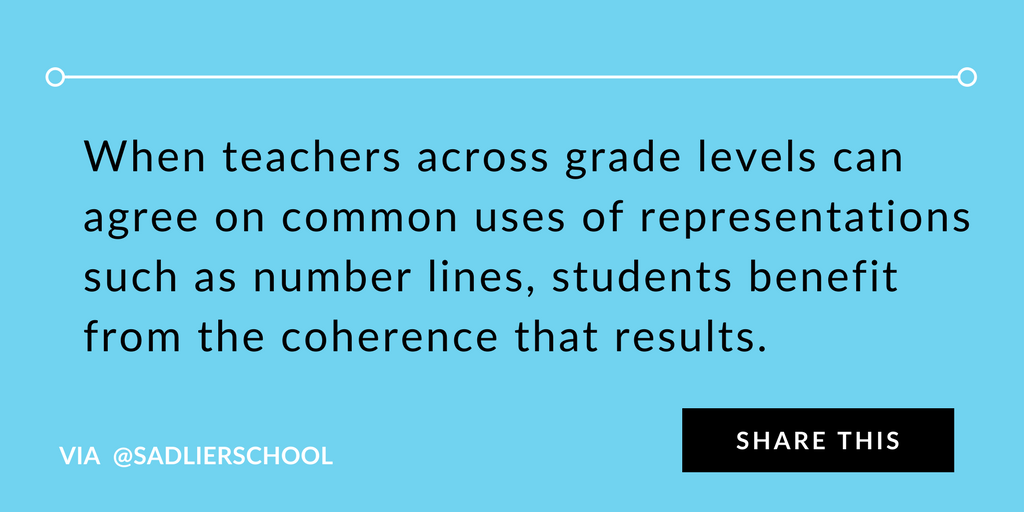1.800.221.5175
Core Program
Full Access
Core Program
Full Access
High-Impact Tutoring
Core Program
Full Access
National Program
Full Access
New York
Critical Thinking for Active Math Minds
Preparing for Standards Based Assessments
Vocabulary Workshop, Tools for Comprehension Grades 1–5
Print Program
Interactive Edition
Print Program
Interactive Edition
Vocabulary Workshop, Tools for Excellence Grades 6–12+
Print Program
Interactive Edition
Vocabulary for Success
Progress English Language Arts Grades K–8
National Program
Full Access
High-Impact Tutoring
New York
Grammar Workshop, Tools for Writing
Grammar for Writing
Print Program
Interactive Practice Bundle
Fluency Booster Practice Book
Interactive Assessments
High-Impact Tutoring
Building Reading Success with Wiley Blevins
Print Program
Interactive Edition
Professional Learning

A K–8 resource to support deep comprehension of math skills and conceptsTopics:

#### December 16, 2019 k-2-math-practices, 3-5-number-and-operations-in-base-10, 3-5-operations-and-algebraic-thinking, 6-8-math-practices, k-2-number-and-operations-in-base-10, other-professional-development, k-2-operations-and-algebraic-thinking, 3-5-number-and-operations-fractions, 3-5-math-practices

Using number lines is one of those topics that are often taken for granted in the elementary and middle school curriculum. Number lines, as a form of representation, help students learn about and explain their developing reasoning about numbers. They allow for coherence across the curriculum, as students deepen their knowledge of the number system and continue to use this familiar representation in increasingly sophisticated ways. The download for this post includes an exhaustive list of ways the number line should be used at each grade level.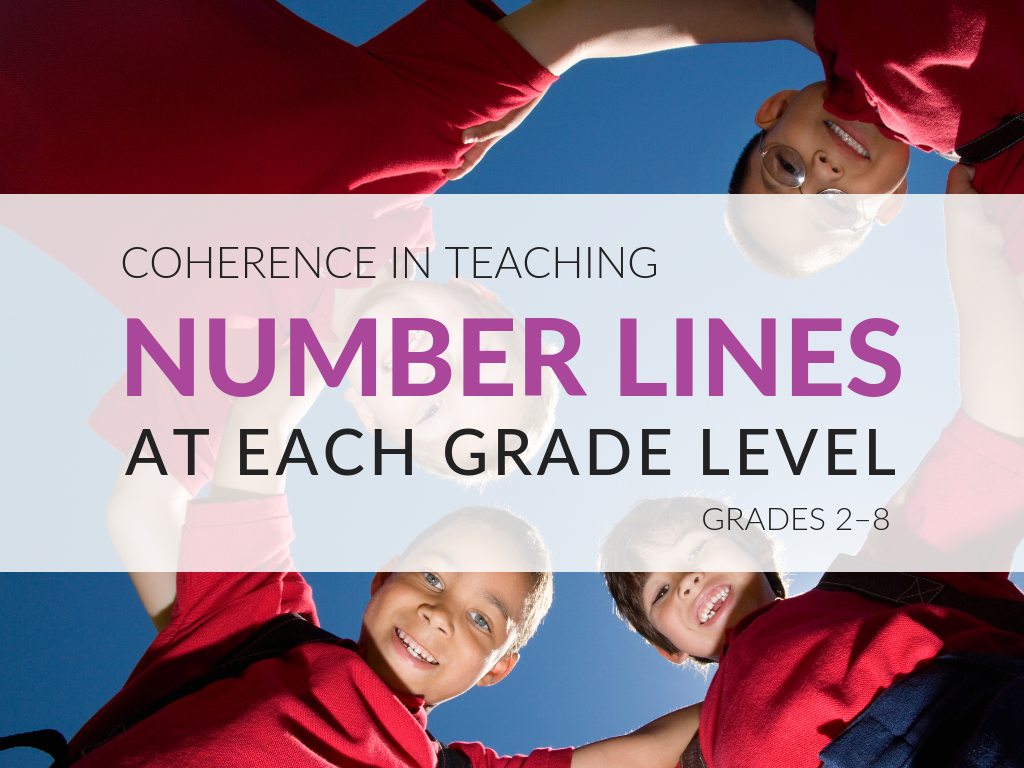My printable list was developed based on the State Standards for Mathematics and using Bill McCallum’s “Progressions” documents that explain the reasoning behind the development of the Common Core State Standards for Mathematics.

It is useful for teachers, math coordinators, coaches, and administrators, so that they can discuss the use of number lines, and will thus know what needs to be taught (and what does not need to be taught) at each grade level.

If you are a grade-level teacher, it can help you understand what came before your grade level and what comes after it. If you are a coach or a supervisor, you can use the guide to initiate cross-grade discussions about the use of number lines in order to create coherence in the curriculum.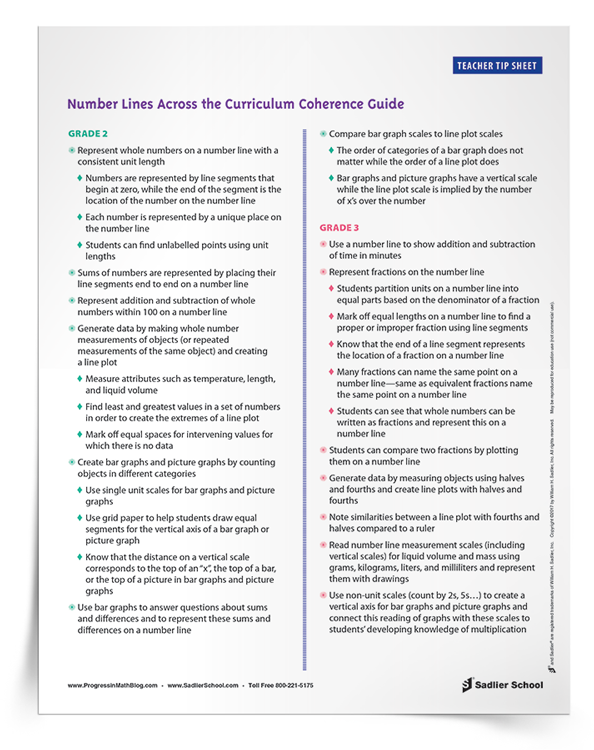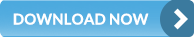## How to Teach Number Lines to Support Math Concepts

Number lines are used for developing the ideas of numbers and operations, for displaying data through the use of scales on graphs, and for reasoning about numbers and their relationships. Tape diagrams and area models could be considered as part of the number line trajectory, but for the purposes of this article are not included.

In addition to these broad topics for number lines, students use number lines to learn about the organization of the number system.

The number line is first used only for whole numbers. In Grades 3 and 4, students begin to use number lines for fractions and operations with fractions. By Grade 6 students are extending the number line to include integers, and are filling in the gaps between integers with rational numbers in Grade 7. The use of the number line culminates in Grade 8 with the introduction of irrational numbers, the last component of the real number system.

## Using Number Lines to Learn About Numbers and Operations

Number lines are a key concept that begins to be used in Grade 2.

The length of a line segment with one end placed at zero and the other end of the segment placed on a number represents the magnitude of that number. The distance between zero and one is a unit length, and that unit length can be placed end to end in order to form longer segments that represent the different whole numbers. This combining of lengths is the operation of addition.

If students can understand this with whole numbers, then using this same representation for unit fractions with the same denominator will be much easier for students. It can then be extended to non-unit fractions and fractions with unlike denominators.

Through the middle school years, directed line segments will build on this foundation, as students learn about integers and other rational numbers.

## Using Number Lines to Display Data

Alongside learning about numbers and operations with numbers, number lines are also used in the display of data from Grade 2 and on for picture graphs, bar graphs, and line plots.

In middle school, more complex data displays are included: histograms and box plots.

In addition, by Grade 5, two perpendicular rays form the first quadrant of the coordinate grid, and students begin graphing ordered pairs. This is extended to quadrants in Grade 6.

By Grade 8, bivariate data are graphed on coordinate grids.

## Using Number Lines to Reason Abstractly About Numbers

One final area in which number lines can be used, which appears in the standards and is worth discussing here, is the use of the number line to reason abstractly about numbers on the number line, using variable names.

This first appears in Grade 6, where students reason about a point “a” on a number line and how to locate its opposite “-a”. In Grade 7, this kind of reasoning is extended to the idea of addition, subtraction, adding the opposite, and distance.

## Number Lines Across the Curriculum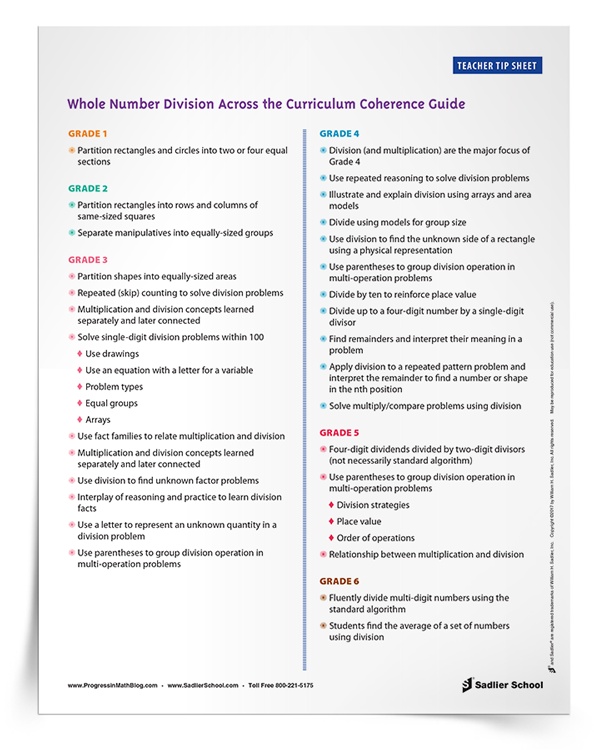Below I've outlined details of how number lines are used in 2nd grade. To get complete details for grades 2–6, download my free tip sheet.

Represent whole numbers on a number line with a consistent unit length
• Numbers are represented by line segments that begin at zero, while the end of the segment is the location of the number on the number line
• Each number is represented by a unique place on the number line
• Students can find unlabelled points using unit lengths

Sums of numbers are represented by placing their line segments end to end on a number line

Represent addition and subtraction of whole numbers within 100 on a number line

Generate data by making whole number measurements of objects (or repeated measurements of the same object) and creating a line plot

• Measure attributes such as temperature, length, and liquid volume
• Find least and greatest values in a set of numbers in order to create the extremes of a line plot
• Mark off equal spaces for intervening values for which there is no data

Create bar graphs and picture graphs by counting objects in different categories

• Use single unit scales for bar graphs and picture graphs
• Use grid paper to help students draw equal segments for the vertical axis of a bar graph or picture graph
• Know that the distance on a vertical scale corresponds to the top of an “x”, the top of a bar, or the top of a picture in bar graphs and picture graphs

Use bar graphs to answer questions about sums and differences and to represent these sums and differences on a number line

Compare bar graph scales to line plot scales

• The order of categories of a bar graph does not matter while the order of a line plot does
• Bar graphs and picture graphs have a vertical scale while the line plot scale is implied by the number of x’s over the number

## In Summary

When teachers across grade levels can agree on common uses of representations such as number lines, students benefit from the coherence that results. Teachers can maximize their impact by focusing on exactly what needs to be taught! Download the Number Line Coherence Guide to better understand what's expected at each grade level.